Robotics 1
Kinematic Diagrams
QuizQuestion 2:  What do we call 'the area the robot can reach'?
Question 3:  We often don't call a robot arm an 'arm', because sometimes it doesn't look very much like an arm.  Instead, what do we call it?
Question 4:  Shown here is a kinematic diagram of a robot.  How many degrees of freedom does it have?
Question 5:  Shown here are four coordinate frames.  One does not follow the right-hand rule.  Which one?
Question 6:  Shown here are four kinematic diagrams.  One has the frames drawn correctly.  Which one?
Question 7:  Shown here are four kinematic diagrams.  Which one has something wrong with it?
Question 8:  Shown here is a kinematic diagram.  Which standard manipulator type is this?
Question 9:  Shown here is a kinematic diagram.  Which standard manipulator type is this?
Question 10: Shown here is a video of a robot manipulator moving around.  Which of these kinematic diagrams is correct for this robot?
Question 11:  Which of the following kinematic diagrams is a spherical wrist?
Question 12:  Only one of these spherical wrist diagrams is labeled correctly.  Which one?
Question 13: A 6-DoF manipulator can be made by attaching a spherical wrist to the end of a standard manipulator type.  Shown here is a kinematic diagram.  Which description matches this diagram?
Question 14:  A 6-DoF manipulator can be made by attaching a spherical wrist to the end of a standard manipulator type.  Shown here is a kinematic diagram.  Which description matches this diagram?
Question 15:  Shown here is a video of a 6-DoF robot manipulator moving around.  Which of these kinematic diagrams is correct for this robot?
Question 1: Only one of these joints has the positive direction of motion labeled incorrectly.  Which one?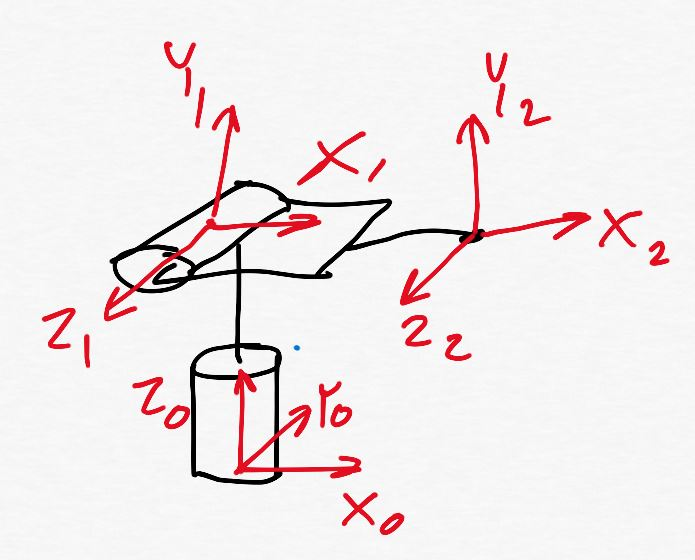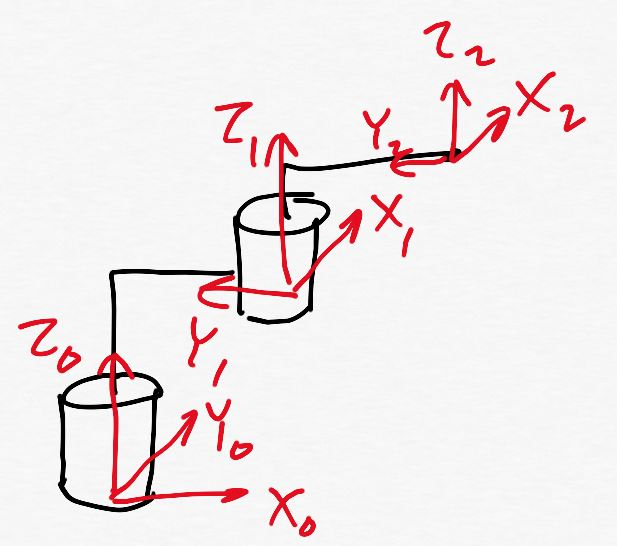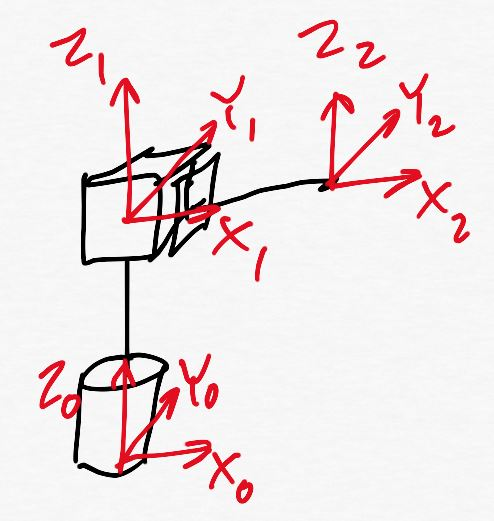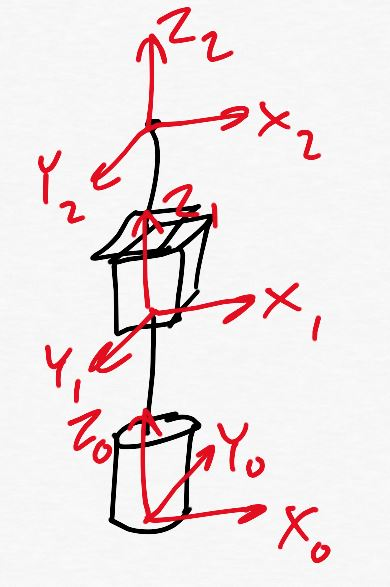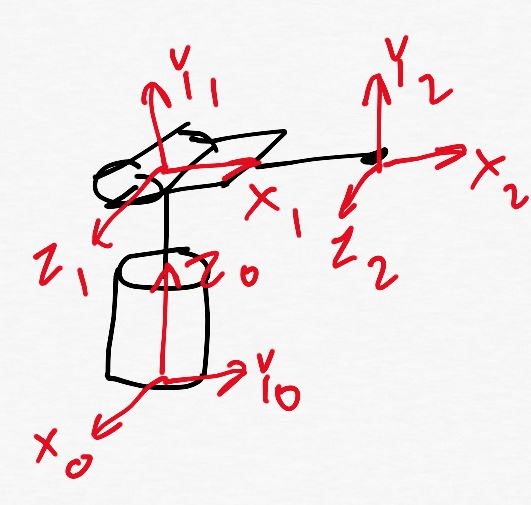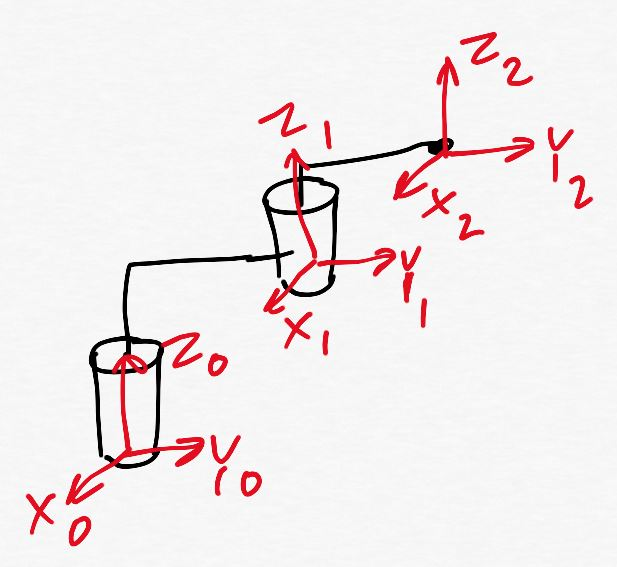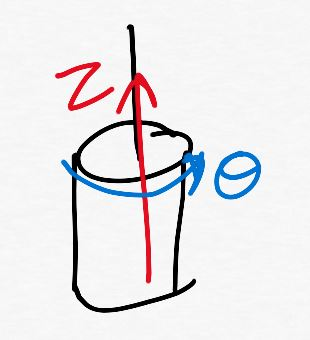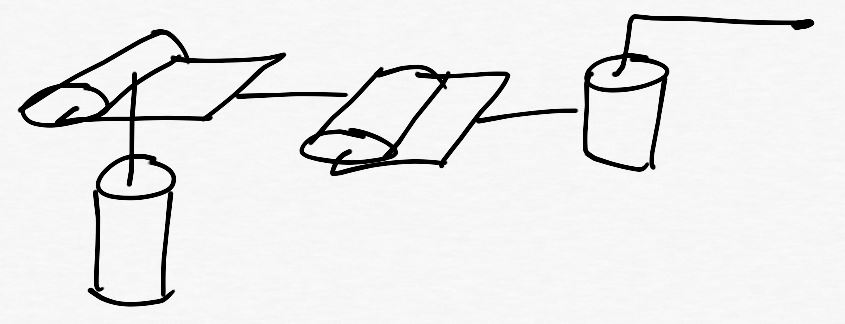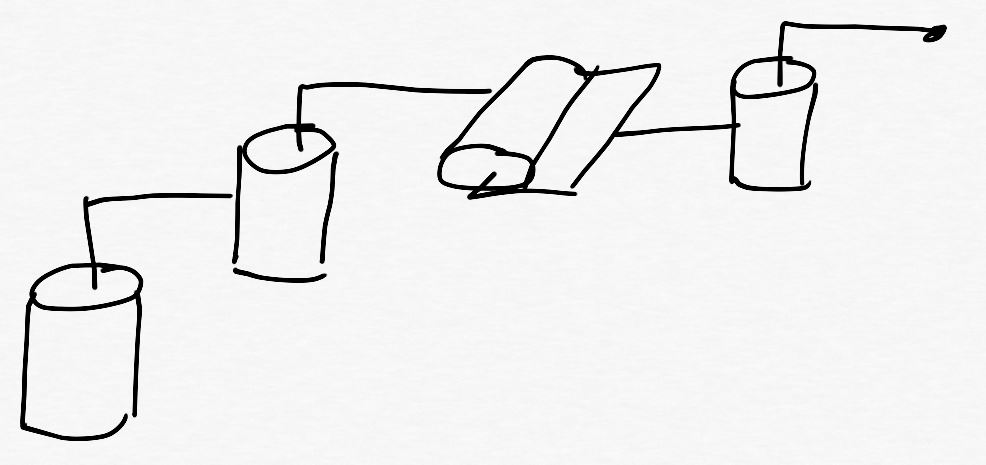Articulated
SCARA
Spherical
Cartesian
CylindricalArticulated
Cartesian
Cylindrical
Spherical
SCARA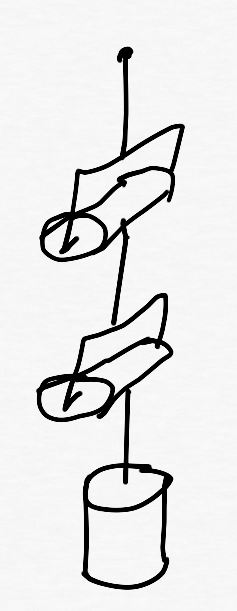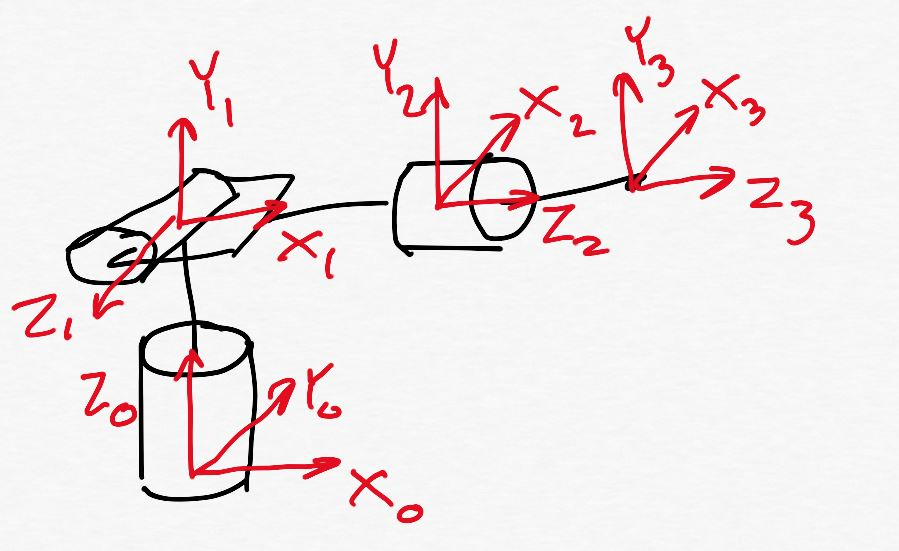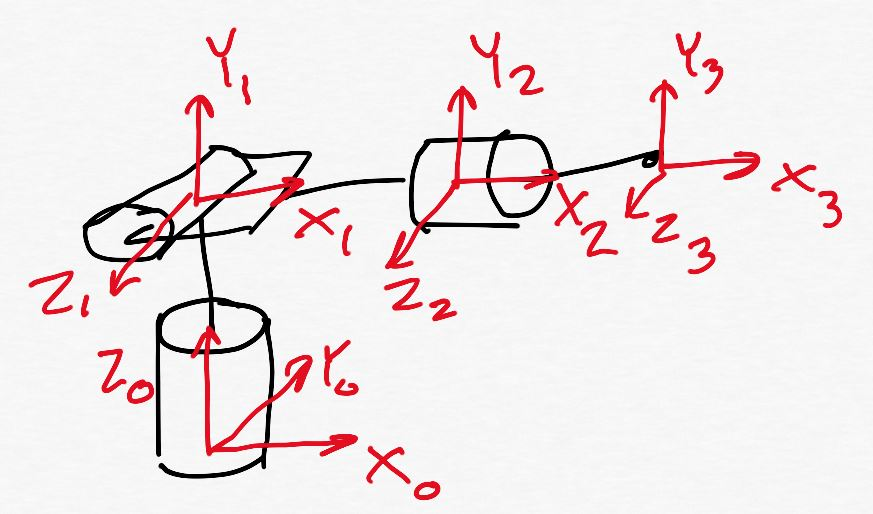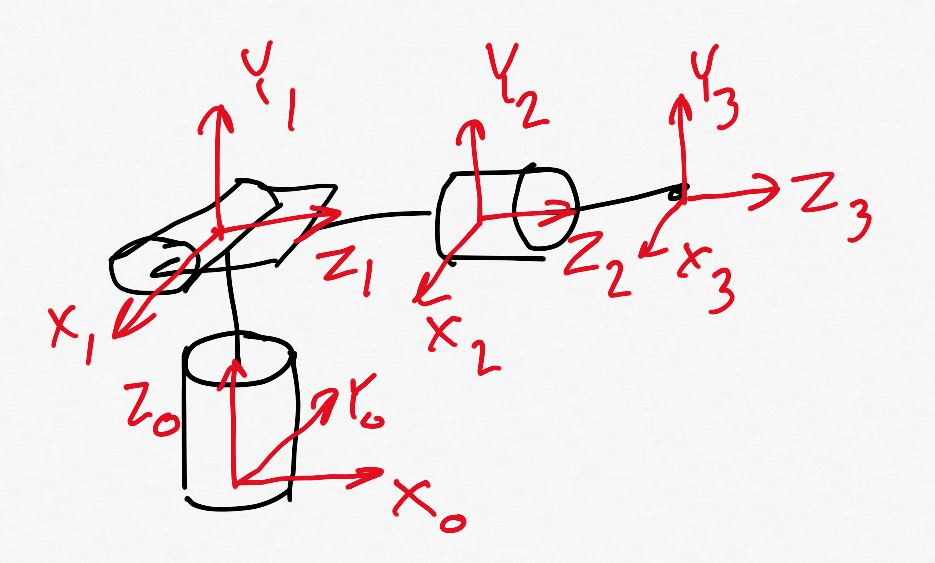Spherical manipulator with a spherical wrist
Cylindrical manipulator with a spherical wrist
SCARA manipulator with a spherical wrist
Articulated manipulator with a spherical wrist
Spherical manipulator with a spherical wrist
Cylindrical manipulator with a spherical wrist
SCARA manipulator with a spherical wrist
Articulated manipulator with a spherical wrist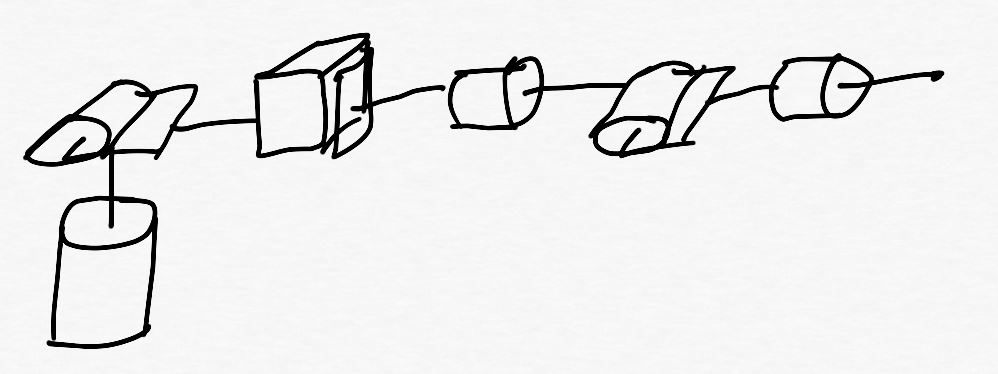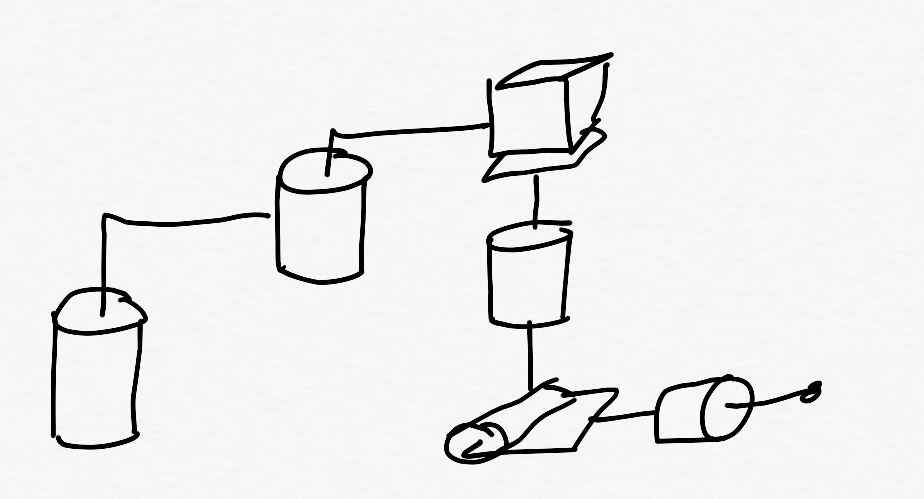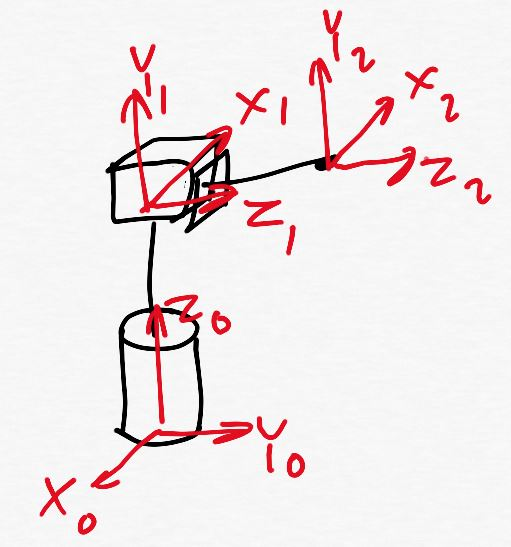1
2
3
4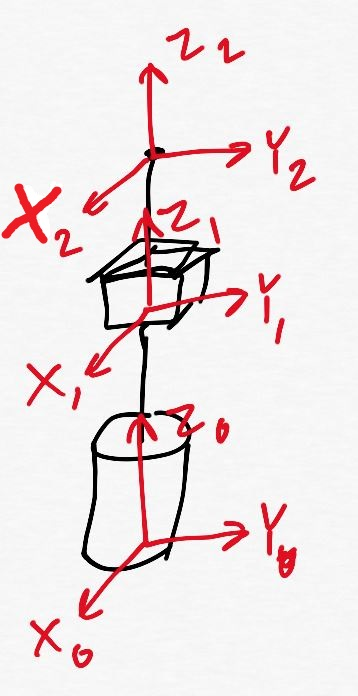The robot range
The robot span
The robot work area
The robot workspace
An end-effector
A manipulator
A gripper
Robot joints